Home » 40 Must know Questions to test a data scientist on Dimensionality Reduction techniques

# 40 Must know Questions to test a data scientist on Dimensionality Reduction techniques

## Introduction

Have you come across a dataset with hundreds of columns and wondered how to build a predictive model on it? Or have come across a situation where a lot of variables might be correlated? It is difficult to escape these situations while working on real life problems.

Thankfully, dimensionality reduction techniques come to our rescue here. Dimensionality Reduction is an important technique in data science. It is a must have skill set for any data scientist. To test your knowledge in dimensionality reduction techniques, we are conducted this skill test. These questions include topics like Principal Component Analysis (PCA), t-SNE and LDA.

Check out more challenging competitions coming up here

A total of 582 people participated in this skill test. The questions varied from theoretical to practical.

If you missed taking the test, here is your opportunity for you to find out how many questions you could have answered correctly.

## Overall Scores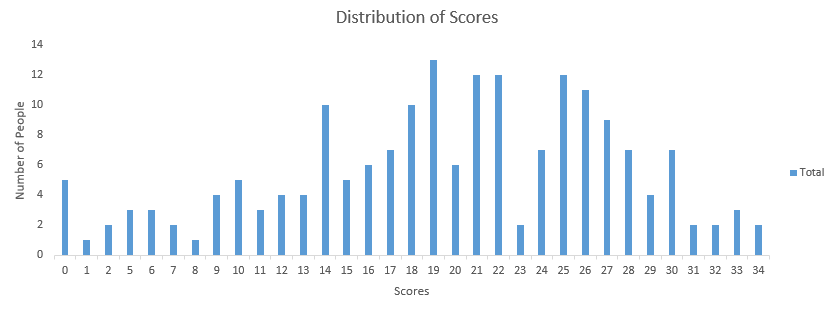You can access your performance here. More than 180 people participated in the skill test and the highest score was 34. Here are a few statistics about the distribution.

Overall distribution

Mean Score: 19.52

Median Score: 20

Mode Score: 19

## Useful Resources

Are you just getting started with Dimensionality Reduction Techniques? Do you want to learn how to use these techniques to work on real-life projects and improve the model performance? Presenting two comprehensive courses which cover all the important concepts like feature selection and dimentionality reduction,-

1) Imagine, you have 1000 input features and 1 target feature in a machine learning problem. You have to select 100 most important features based on the relationship between input features and the target features.

Do you think, this is an example of dimensionality reduction?

A. Yes

B. No

2) [ True or False ] It is not necessary to have a target variable for applying dimensionality reduction algorithms.

A. TRUE

B. FALSE

3) I have 4 variables in the dataset such as – A, B, C & D. I have performed the following actions:

Step 1: Using the above variables, I have created two more variables, namely E = A + 3 * B and F = B + 5 * C + D.

Step 2: Then using only the variables E and F I have built a Random Forest model.

Could the steps performed above represent a dimensionality reduction method?

A. True

B. False

4) Which of the following techniques would perform better for reducing dimensions of a data set?

A. Removing columns which have too many missing values

B. Removing columns which have high variance in data

C. Removing columns with dissimilar data trends

D. None of these

5) [ True or False ] Dimensionality reduction algorithms are one of the possible ways to reduce the computation time required to build a model.

A. TRUE

B. FALSE

6) Which of the following algorithms cannot be used for reducing the dimensionality of data?

A. t-SNE

B. PCA

C. LDA False

D. None of these

Solution: (D)

All of the algorithms are the example of dimensionality reduction algorithm.

7) [ True or False ] PCA can be used for projecting and visualizing data in lower dimensions.

A. TRUE

B. FALSE

Solution: (A)

Sometimes it is very useful to plot the data in lower dimensions. We can take the first 2 principal components and then visualize the data using scatter plot.

8) The most popularly used dimensionality reduction algorithm is Principal Component Analysis (PCA). Which of the following is/are true about PCA?

1. PCA is an unsupervised method
2. It searches for the directions that data have the largest variance
3. Maximum number of principal components <= number of features
4. All principal components are orthogonal to each other

A. 1 and 2

B. 1 and 3

C. 2 and 3

D. 1, 2 and 3

E. 1,2 and 4

F. All of the above

Solution: (F)

All options are self explanatory.

9) Suppose we are using dimensionality reduction as pre-processing technique, i.e, instead of using all the features, we reduce the data to k dimensions with PCA. And then use these PCA projections as our features. Which of the following statement is correct?

A. Higher ‘k’ means more regularization

B. Higher ‘k’ means less regularization

C. Can’t Say

Solution: (B)

Higher k would lead to less smoothening as we would be able to preserve more characteristics in data, hence less regularization.

10) In which of the following scenarios is t-SNE better to use than PCA for dimensionality reduction while working on a local machine with minimal computational power?

A. Dataset with 1 Million entries and 300 features

B. Dataset with 100000 entries and 310 features

C. Dataset with 10,000 entries and 8 features

D. Dataset with 10,000 entries and 200 features

Solution: (C)

t-SNE has quadratic time and space complexity. Thus it is a very heavy algorithm in terms of system resource utilization.

11) Which of the following statement is true for a t-SNE cost function?

A. It is asymmetric in nature.

B. It is symmetric in nature.

C. It is same as the cost function for SNE.

Solution: (B)

Cost function of SNE is asymmetric in nature. Which makes it difficult to converge using gradient decent. A symmetric cost function is one of the major differences between SNE and t-SNE.

Question 12

Imagine you are dealing with text data. To represent the words you are using word embedding (Word2vec). In word embedding, you will end up with 1000 dimensions. Now, you want to reduce the dimensionality of this high dimensional data such that, similar words should have a similar meaning in nearest neighbor space.In such case, which of the following algorithm are you most likely choose?

A. t-SNE

B. PCA

C. LDA

D. None of these

Solution: (A)

t-SNE stands for t-Distributed Stochastic Neighbor Embedding which consider the nearest neighbours for reducing the data.

13) [True or False] t-SNE learns non-parametric mapping.

A. TRUE

B. FALSE

Solution: (A)

t-SNE learns a non-parametric mapping, which means that it does not learn an explicit function that maps data from the input space to the map. For more information read from this link.

14) Which of the following statement is correct for t-SNE and PCA?

A. t-SNE is linear whereas PCA is non-linear

B. t-SNE and PCA both are linear

C. t-SNE and PCA both are nonlinear

D. t-SNE is nonlinear whereas PCA is linear

Solution: (D)

15) In t-SNE algorithm, which of the following hyper parameters can be tuned?

A. Number of dimensions

B. Smooth measure of effective number of neighbours

C. Maximum number of iterations

D. All of the above

Solution: (D)

All of the hyper-parameters in the option can tuned.

16) What is of the following statement is true about t-SNE in comparison to PCA?

A. When the data is huge (in size), t-SNE may fail to produce better results.

B. T-NSE always produces better result regardless of the size of the data

C. PCA always performs better than t-SNE for smaller size data.

D. None of these

Solution: (A)

Option A is correct

17) Xi and Xj are two distinct points in the higher dimension representation, where as Yi & Yj are the representations of Xi and Xj in a lower dimension.

1. The similarity of datapoint Xi to datapoint Xj is the conditional probability p (j|i) .

2. The similarity of datapoint Yi to datapoint Yj is the conditional probability q (j|i) .

Which of the following must be true for perfect representation of xi and xj in lower dimensional space?

A. p (j|i) = 0 and q (j|i) = 1

B. p (j|i) < q (j|i)

C. p (j|i) = q (j|i)

D. p (j|i) > q (j|i)

Solution: (C)

The conditional probabilities for similarity of two points must be equal because similarity between the points must remain unchanged in both higher and lower dimension for them to be perfect representations.

18) Which of the following is true about LDA?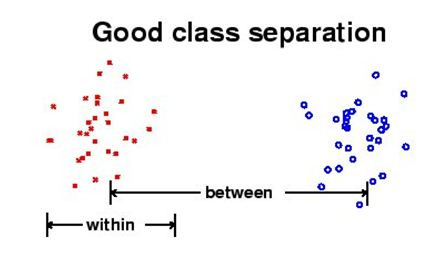A. LDA aims to maximize the distance between class and minimize the within class distance

B. LDA aims to minimize both distance between class and distance within class

C. LDA aims to minimize the distance between class and maximize the distance within class

D. LDA aims to maximize both distance between class and distance within class

Solution: (A)

Option A is correct.

19) In which of the following case LDA will fail?

A. If the discriminatory information is not in the mean but in the variance of the data

B. If the discriminatory information is in the mean but not in the variance of the data

C. If the discriminatory information is in the mean and variance of the data

D. None of these

Solution: (A)

Option A is correct

20) Which of the following comparison(s) are true about PCA and LDA?

1. Both LDA and PCA are linear transformation techniques
2. LDA is supervised whereas PCA is unsupervised
3. PCA maximize the variance of the data, whereas LDA maximize the separation between different classes,

A. 1 and 2

B. 2 and 3

C. 1 and 3

D. Only 3

E. 1, 2 and 3

Solution: (E)

All of the options are correct

21) What will happen when eigenvalues are roughly equal?

A. PCA will perform outstandingly

C. Can’t Say

D.None of above

Solution: (B)

When all eigen vectors are same in such case you won’t be able to select the principal components because in that case all principal components are equal.

22) PCA works better if there is?

1. A linear structure in the data
2. If the data lies on a curved surface and not on a flat surface
3. If variables are scaled in the same unit

A. 1 and 2

B. 2 and 3

C. 1 and 3

D. 1 ,2 and 3

Solution: (C)

Option C is correct

23) What happens when you get features in lower dimensions using PCA?

1. The features will still have interpretability
2. The features will lose interpretability
3. The features must carry all information present in data
4. The features may not carry all information present in data

A. 1 and 3

B. 1 and 4

C. 2 and 3

D. 2 and 4

Solution: (D)

When you get the features in lower dimensions then you will lose some information of data most of the times and you won’t be able to interpret the lower dimension data.

24) Imagine, you are given the following scatterplot between height and weight.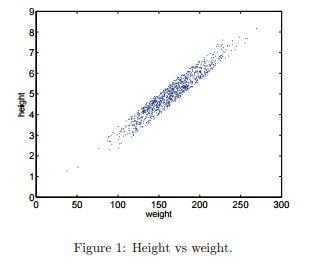Select the angle which will capture maximum variability along a single axis?

A. ~ 0 degree

B. ~ 45 degree

C. ~ 60 degree

D. ~ 90 degree

Solution: (B)

Option B has largest possible variance in data.

25) Which of the following option(s) is / are true?

1. You need to initialize parameters in PCA
2. You don’t need to initialize parameters in PCA
3. PCA can be trapped into local minima problem
4. PCA can’t be trapped into local minima problem

A. 1 and 3

B. 1 and 4

C. 2 and 3

D. 2 and 4

Solution: (D)

PCA is a deterministic algorithm which doesn’t have parameters to initialize and it doesn’t have local minima problem like most of the machine learning algorithms has.

Question Context 26

The below snapshot shows the scatter plot of two features (X1 and X2) with the class information (Red, Blue). You can also see the direction of PCA and LDA.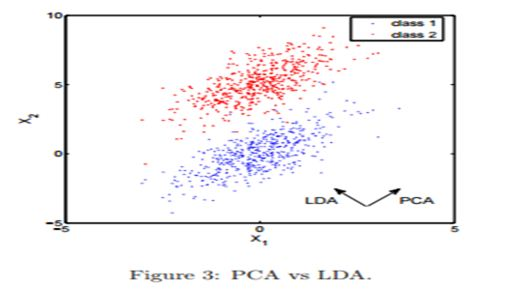26) Which of the following method would result into better class prediction?

A. Building a classification algorithm with PCA (A principal component in direction of PCA)

B. Building a classification algorithm with LDA

C. Can’t say

D. None of these

Solution: (B)

If our goal is to classify these points, PCA projection does only more harm than good—the majority of blue and red points would land overlapped on the first principal component.hence PCA would confuse the classifier.

27) Which of the following options are correct, when you are applying PCA on a image dataset?

1. It can be used to effectively detect deformable objects.
2. It is invariant to affine transforms.
3. It can be used for lossy image compression.
4. It is not invariant to shadows.

A. 1 and 2

B. 2 and 3

C. 3 and 4

D. 1 and 4

Solution: (C)

Option C is correct

28) Under which condition SVD and PCA produce the same projection result?

A. When data has zero median

B. When data has zero mean

C. Both are always same

D. None of these

Solution: (B)

When the data has a zero mean vector, otherwise you have to center the data first before taking SVD.

Question Context 29

Consider 3 data points in the 2-d space: (-1, -1), (0,0), (1,1).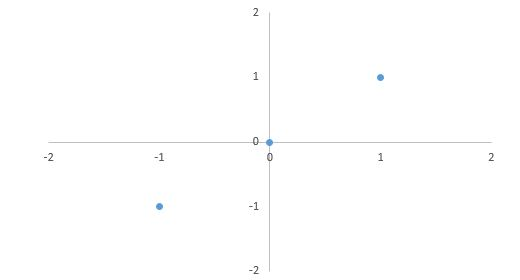29) What will be the first principal component for this data?

1. [ √ 2 /2 , √ 2/ 2 ]
2. (1/ √ 3, 1/ √ 3)
3. ([ -√ 2/ 2 , √ 2/ 2 ])
4. (- 1/ √ 3, – 1/ √ 3)

A. 1 and 2

B. 3 and 4

C. 1 and 3

D. 2 and 4

Solution: (C)

The first principal component is v = [ √ 2 /2 , √ 2/ 2 ] T (you shouldn’t really need to solve any SVD or eigenproblem to see this). Note that the principal component should be normalized to have unit length. (The negation v = [− √ 2/ 2 , − √ 2/ 2 ] T is also correct.)

30) If we project the original data points into the 1-d subspace by the principal component [ √ 2 /2, √ 2 /2 ] T. What are their coordinates in the 1-d subspace?

A. (− √ 2 ), (0), (√ 2)

B. (√ 2 ), (0), (√ 2)

C. ( √ 2 ), (0), (-√ 2)

D. (-√ 2 ), (0), (-√ 2)

Solution: (A)

The coordinates of three points after projection should be z1 = x T 1 v = [−1, −1][ √ 2/ 2 , √ 2 /2 ] T = − √ 2, z2 = x T 2 v = 0, z3 = x T 3 v = √ 2.

31) For the projected data you just obtained projections ( (− √ 2 ), (0), (√ 2) ). Now if we represent them in the original 2-d space and consider them as the reconstruction of the original data points, what is the reconstruction error? Context: 29-31:

A. 0%

B. 10%

C. 30%

D. 40%

Solution: (A)

The reconstruction error is 0, since all three points are perfectly located on the direction of the first principal component. Or, you can actually calculate the reconstruction: z1 ·v.

xˆ1 = − √ 2·[ √ 2/ 2 , √ 2/2 ] T = [−1, −1]T
xˆ2 = 0*[0, 0]T = [0,0]
xˆ3 = √ 2* [1, 1]T = [1,1]

which are exactly x1, x2, x3.

32) In LDA, the idea is to find the line that best separates the two classes. In the given image which of the following is a good projection?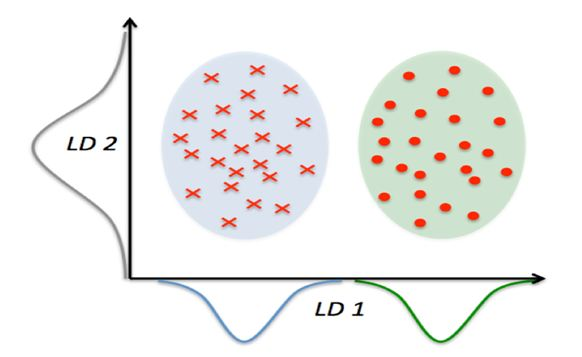A. LD1

B. LD2

C. Both

D. None of these

Solution: (A)

LD1 Is a good projection because it best separates the class.

Question Context 33

PCA is a good technique to try, because it is simple to understand and is commonly used to reduce the dimensionality of the data. Obtain the eigenvalues λ1 ≥ λ2 ≥ • • • ≥ λN and plot.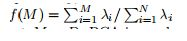To see how f(M) increases with M and takes maximum value 1 at M = D. We have two graph given below: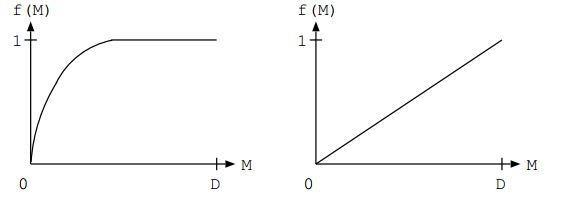33) Which of the above graph shows better performance of PCA? Where M is first M principal components and D is total number of features?

A. Left

B. Right

C. Any of A and B

D. None of these

Solution: (A)

PCA is good if f(M) asymptotes rapidly to 1. This happens if the first eigenvalues are big and the remainder are small. PCA is bad if all the eigenvalues are roughly equal. See examples of both cases in figure.

34) Which of the following option is true?

A. LDA explicitly attempts to model the difference between the classes of data. PCA on the other hand does not take into account any difference in class.

B. Both attempt to model the difference between the classes of data.

C. PCA explicitly attempts to model the difference between the classes of data. LDA on the other hand does not take into account any difference in class.

D. Both don’t attempt to model the difference between the classes of data.

Solution: (A)

Options are self explanatory.

35) Which of the following can be the first 2 principal components after applying PCA?

1. (0.5, 0.5, 0.5, 0.5) and (0.71, 0.71, 0, 0)
2. (0.5, 0.5, 0.5, 0.5) and (0, 0, -0.71, -0.71)
3. (0.5, 0.5, 0.5, 0.5) and (0.5, 0.5, -0.5, -0.5)
4. (0.5, 0.5, 0.5, 0.5) and (-0.5, -0.5, 0.5, 0.5)

A. 1 and 2

B. 1 and 3

C. 2 and 4

D. 3 and 4

Solution: (D)

For the first two choices, the two loading vectors are not orthogonal.

36) Which of the following gives the difference(s) between the logistic regression and LDA?

1. If the classes are well separated, the parameter estimates for logistic regression can be unstable.
2. If the sample size is small and distribution of features are normal for each class. In such case, linear discriminant analysis is more stable than logistic regression.

A. 1

B. 2

C. 1 and 2

D. None of these

Solution: (C)

Refer this video

37) Which of the following offset, do we consider in PCA?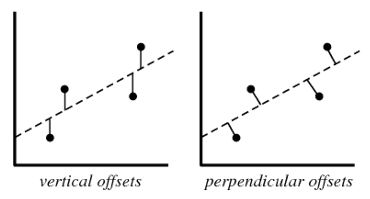A. Vertical offset

B. Perpendicular offset

C. Both

D. None of these

Solution: (B)

We always consider residual as vertical offsets. Perpendicular offset are useful in case of PCA

38) Imagine you are dealing with 10 class classification problem and you want to know that at most how many discriminant vectors can be produced by LDA. What is the correct answer?

A. 20

B. 9

C. 21

D. 11

E. 10

Solution: (B)

LDA produces at most c − 1 discriminant vectors. You may refer this link for more information.

Question Context 39

The given dataset consists of images of “Hoover Tower” and some other towers. Now, you want to use PCA (Eigenface) and the nearest neighbour method to build a classifier that predicts whether new image depicts “Hoover tower” or not. The figure gives the sample of your input training images.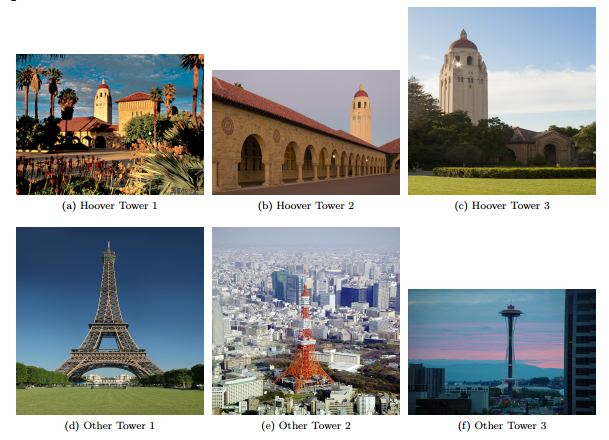39) In order to get reasonable performance from the “Eigenface” algorithm, what pre-processing steps will be required on these images?

1. Align the towers in the same position in the image.
2. Scale or crop all images to the same size.

A. 1

B. 2

C. 1 and 2

D. None of these

Solution: (C)

Both the statements are correct.

40) What are the optimum number of principle components in the below figure ?

B. 30

C. 40

D. Can’t Say

Solution: (B)

We can see in the above figure that the number of components = 30 is giving highest variance with lowest number of components. Hence option ‘B’ is the right answer.

### End Notes

I hope you enjoyed taking the test and found the solutions helpful. The test focused on conceptual as well as practical knowledge of dimensionality reduction.

If you have any doubts in the questions above, let us know through comments below. Also, If you have any suggestions or improvements you think we should make in the next skill test, you can let us know by dropping your feedback in the comments section. Also, checkout DATAFEST 2017.

## Learn, compete, hack and get hired!

You can also read this article on our Mobile APP•Pratima Joshi says:

Hi,
I think the answers and explanations of questions 10 and 11 are not in sync. Please revisit and correct.

•Ankit Gupta says:

Hi Pratima,

Thanks for noticing!

I change the explanation of question number 10 which was addressing some other issue. Answers for questions 10 and 11 are remain same

Best Regards,
Ankit Gupta

•Marvin says:

Hi ,
could it be that in question 33 solution and explanation are contradicting or did I get it wrong?

•Ankit Gupta says:

Hi Marvin,

Explanation is correct but solution was incorrectly marked. Thanks for noticing

Best!
Ankit Gupta

• […] 查看原文>>> […]

•Keyuri says:

Answer for the Q.20 should be C because both LDA and PCA are unsupervised methods.